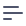# Polymorphic Cubes

In programming languages, polymorphism usually means the use of a single symbol to represent multiple different types. It can be quite common for a database and application to be designed in such a way that leverages a single database table for entities of different types that share common traits.

For example, you are working on an online education platform, where teachers assign lessons to students. The database can contain only two tables: one for users and another one for lessons. The users table can contain a type column, with possible values teacher or student. Here is how it could look:

idtypenameschool
1studentCarl AndersonBalboa High School
2studentLuke SkywalkerBalboa High School
31teacherJohn DoeBalboa High School

Lessons are assigned by teachers and completed by students. The lessons table has both teacher_id and student_id, which are actually references to the user id. The lessons table can look like this:

idteacher_idstudent_idname
100311Multiplication and the meaning of the Factors
101312Division as an Unknown Factor Problem

The best way to design such a schema is by using what we call Polymorphic Cubes. It relies on the extends feature and prevents you from duplicating code, while preserving the correct domain logic. Learn more about using extends here.

The first step is to create a User cube, which will act as a base cube for our Teachers and Students cubes and will contain all common measures and dimensions:

cube(Users, {
sql: SELECT * FROM USERS,

measures: {
count: {
type: count,
},
},

dimensions: {
name: {
sql: name,
type: string,
},

school: {
sql: school,
type: string,
},
},
});

Then you can extend the Teachers and Students cubes from Users:

cube(Teachers, {
extends: Users,
sql: SELECT * FROM ${Users.sql()} WHERE type = 'teacher', }); cube(Students, { extends: Users, sql: SELECT * FROM${Users.sql()} WHERE type = 'student',
});

Once we have those cubes, we can define correct joins from the Lessons cube:

cube(Lessons, {
sql: SELECT * FROM LESSONS,

joins: {
Students: {
relationship: belongsTo,
sql: ${CUBE}.student_id =${Students.id},
},
Teachers: {
relationship: belongsTo,
sql: ${CUBE}.teacher_id =${Teachers.id},
},
},
});